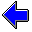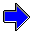Java Security Settings:
This web page employs Java, which requires specific security settings for correct operation.
If the applets on this page do not run correctly, consult the Virtual Chemistry Experiments FAQ
or the Physlet Physics web site for establishing the correct security settings.

# Kinetic Molecular Theory

## Maxwell Distribution

### Concepts

In the context of the Kinetic Molecular Theory of Gases, a gas contains a large number of particles in rapid motions. Each particle has a different speed, and each collision between particles changes the speeds of the particles. An understanding of the properties of the gas requires an understanding of the distribution of particle speeds.

The simulation below implements the behavior postulated by the Kinetic Molecular Theory. The histogram at the right shows the distribution of speeds. The speed range (along the horizontal axis) of 0 to 5000 m/sec is divided into 50 cells (0 to 100 m/sec, 100 to 200 m/sec, etc.). The height of the bar for a particular cell equals the cumulative number of particles having a speed in that cell's range. The histogram is updated frequently, and the height of all of the bars increases with time.

Run the simulation for awhile until the shape of the speed distribution becomes well-defined. What is the shape of the distribution? Is it symmetric (as many slow-moving particles as fast-moving particles)? How fast are the particles moving?

After starting the simulation, it takes a few second for the collection of particles in the simulation to equilibrate. The histogram may flicker or be blank during this period.

### Properties of the Maxwell Distribution

The Maxwell distribution describes the distribution of particle speeds in an ideal gas. The distribution may be characterized in a variety of ways.

• Average Speed
The average speed is the sum of the speeds of all of the particles divided by the number of particles.
• Most Probable Speed
The most probable speed is the speed associated with the highest point in the Maxwell distribution. Only a small fraction of particles might have this speed, but it is more likely than any other speed.
• Width of the Distribution
The width of the distribution characterizes the most likely range of speeds for the particles. One measure of the width is the Full Width at Half Maximum (FWHM). To determine this value, find the height of the distribution at the most probable speed (this is the maximum height of the distribution). Divide the maximum height by two to obtain the half height, and locate the two speeds in the distribution that have this half-height value. On speed will be greater than the most probably speed and the other speed will be smaller. The full width is the difference between the two speeds at the half-maximum value.

The simulation below depicts the behavior of 100 helium atoms at five different temperatures. Run the simulation at each temperature sufficiently long to obtain a reasonably well-defined distribution of speeds.

At each temperature measure (1) the most probably speed and (2) the full width at half-maximum (FWHM). Owing to the low resolution of the histogram, these values will be approximate.

What happens to the shape of the Maxwell distribution as the temperature increases?

Carefully observe the behavior of the particles at each temperature. How is the particle behavior reflected in the histogram?Basic Concepts of the Kinetic Molecular Theory                     Pressure of GasKinetic Molecular Theory Home PageVirtual Chemistry Home Page

Maxwell.html version 1.3